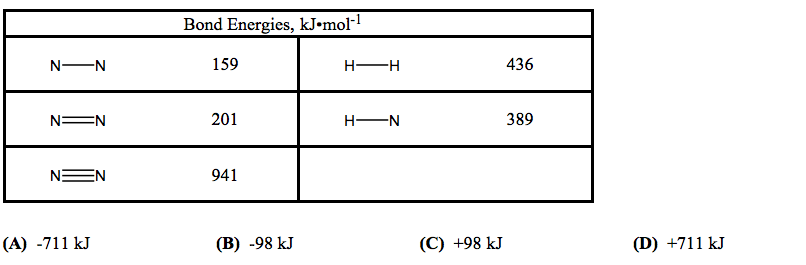# Problem: Use the bond energies in the table to determine  ΔH for the formation of hydrazine, N2H4, from nitrogen and hydrogen according to this equation:N2(g) + 2H2(g) → N2H4(g)

###### FREE Expert Solution
87% (480 ratings)
###### Problem Details

Use the bond energies in the table to determine  ΔH for the formation of hydrazine, N2H4, from nitrogen and hydrogen according to this equation:

N2(g) + 2H2(g) → N2H4(g)What scientific concept do you need to know in order to solve this problem?

Our tutors have indicated that to solve this problem you will need to apply the Bond Energy concept. You can view video lessons to learn Bond Energy. Or if you need more Bond Energy practice, you can also practice Bond Energy practice problems.

What is the difficulty of this problem?

Our tutors rated the difficulty ofUse the bond energies in the table to determine  ΔH for the ...as medium difficulty.

How long does this problem take to solve?

Our expert Chemistry tutor, Sabrina took 7 minutes and 35 seconds to solve this problem. You can follow their steps in the video explanation above.

What professor is this problem relevant for?

Based on our data, we think this problem is relevant for Professor Tang's class at USF.